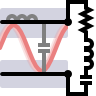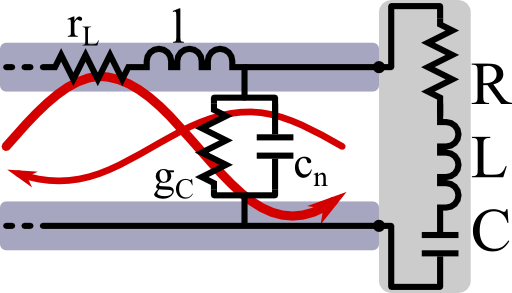Impedance Matching of Transmission Lines and Oscillator CircuitsFor technical applications it is extremely important to be able to transfer as much energy from a transmission line to some load. In this problem we will learn how this is achieved and understand the basic principles signal reflection and impedance matching.

Problem StatementFind out how to impedance-match a serial oscillator circuit to a transmission line! Proceed as follows:

1. In The Transmission Line - Deriving the Telegrapher Equation we found that a transmission line is described by the telegrapher equation$\begin{eqnarray*} \partial_{xx}U\left(x,\omega\right)&=&\left(r_{L}-\mathrm{i}\omega l\right)\left(g_{C}-\mathrm{i}\omega c\right)U\left(x,\omega\right)\\&\equiv&-k^{2}\left(\omega\right)U\left(x,\omega\right)\end{eqnarray*}$which also holds for the current. Here, the parameters are quantities per length. In frequency space, the telegrapher equation is a one dimensional Helmholtz equation which permits propagating solutions of the form$\begin{eqnarray*} U\left(x,\omega\right)&=&U_{0}^{+}\left(\omega\right)e^{\mathrm{i}k\left(\omega\right)x}+ U_{0}^{-}\left(\omega\right)e^{-\mathrm{i}k\left(\omega\right)x} \end{eqnarray*}$where now the $$U_{0}^{\pm}$$ are modal amplitudes. The characteristic impedance is the ratio between voltage and current for outwards propagating waves, $$Z_{0}\left(\omega\right)=U_{0}^{+}\left(\omega\right)/I_{0}^{+}\left(\omega\right)$$. Using the relations between voltage and current, calculate the characteristic impedance for the transmission line.
2. Find the general voltage reflection coefficient $$\Gamma\left(\omega\right)=U_{0}^{-}\left(\omega\right)/U_{0}^{+}\left(\omega\right)$$ if the transmission line is terminated at $$x=0$$ and attached to some load with impedance $$Z_{L}\left(\omega\right)=U_{L}\left(\omega\right)/I_{L}\left(\omega\right)$$. Show under which condition a serial $$RLC$$-circuit attached to a lossless transmission line with $$g_{C}=r_{L}=0$$ is impedance matched, i.e. can absorb all of the incoming energy.